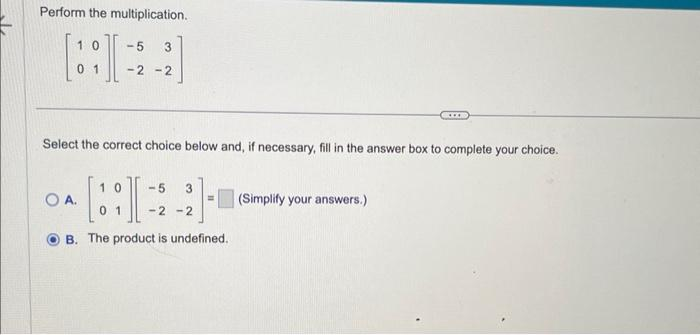Home / Expert Answers / Other Math / 13-perform-the-multiplication-left-begin-array-ll-1-0-0-1-end-array-right-left-be-pa534

# (Solved): 13 Perform the multiplication. $\left[\begin{array}{ll} 1 & 0 \\ 0 & 1 \end{array}\right]\left[\be ... 13Perform the multiplication. \[ \left[\begin{array}{ll} 1 & 0 \\ 0 & 1 \end{array}\right]\left[\begin{array}{rr} -5 & 3 \\ -2 & -2 \end{array}\right]$ Select the correct choice below and, if necessary, fill in the answer box to complete your choice. A. $$\left[\begin{array}{ll}1 & 0 \\ 0 & 1\end{array}\right]\left[\begin{array}{rr}-5 & 3 \\ -2 & -2\end{array}\right]=\quad$$ (Simplify your answers.) B. The product is undefined.

We have an Answer from Expert